# Oops...

This website is made for modern browsers. You are seeing this, because your browser is missing a feature or two. Please install the latest update or switch to a modern browser. See you soon.

# Space frame roof

In this tutorial, we will create a typical space frame roof and continue to get familiar with Grasshopper. You can read more about the structural idea of space frames on this page

.

Planar space frames are typically created by combining different types of polyhedra. For the following exercise we choose the combination of two platonic solids: Half-octahedron and tetrahedron (see figure below). Alternatively, we can imagine the space frame as two parallel, mutually shifted square grids, which are separated from each other by the distance $h$ and whose nodes are connected by spatial diagonals.

In this tutorial, we set the base length $a = 1$ and thus the distance is $h = 1 / 2 \cdot \sqrt 2 = 0,707$.

## Grasshopper

#### 1

##### Generate top grid

First thing to do is to create a grid. We do this by placing the component Square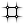Square (SqGrid)
Vector  >  Grid  >  Square
2D grid with square cells
Inputs
Plane (P)Base plane for grid
Size (S)Size of grid cells
Extent X (Ex)Number of grid cells in base plane x direction
Extent Y (Ey)Number of grid cells in base plane y direction
Outputs
Cells (C)Grid cell outlines
Points (P)Points at grid corners
on the canvas. We could use input S to define a cell size, but this component uses already the desired value 1 as its default value.
We can see the preset value by hovering the input grip with the mouse cursor.
We can see the preset value by hovering the input grip with the mouse cursor.

The inputs Ex and Ey determine the number of cells that are generated in the respective direction of the square grid. If we type "10 into the canvas search, we get a PanelPanel
Params  >  Input  >  Panel
A panel for custom notes and text values
with the value 10. After connecting this panel to the inputs Ex and Ey, a grid with 10 × 10 squares will be created.

Input P takes a construction plane as input. Here, the default value is World XY, which is a plane that is defined in x- and y-direction and whose origin is has the coordinates 0,0,0. We get the same result, if we connect an XY Plane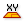XY Plane (XY)
Vector  >  Plane  >  XY Plane
World XY plane.
Inputs
Origin (O)Origin of plane
Outputs
Plane (P)World XY plane
to input P.

#### 2

##### Define origin of bottom grid

Now that we have created one grid, we will create a second one that is offset to the first grid. To do this, we create a construction plane with a new point of origin; the component Construct Point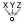Construct Point (Pt)
Vector  >  Point  >  Construct Point
Construct a point from {xyz} coordinates.
Inputs
X coordinate (X){x} coordinate
Y coordinate (Y){y} coordinate
Z coordinate (Z){z} coordinate
Outputs
Point (Pt)Point coordinate
will create this point with the coordinates that are given at the three inputs.

The horizontal offset of the grids is half of the cell size. Therefore, we create a PanelPanel
Params  >  Input  >  Panel
A panel for custom notes and text values
with the value 0.5 and connect it to the inputs X and Y of Construct Point. The vertical offset is $h = 0.707$ and the second grid should be below the first one. Accordingly, we create another PanelPanel
Params  >  Input  >  Panel
A panel for custom notes and text values
with -0.707 and connect it to input Z.

#### 3

##### Generate bottom grid

The second grid is also created with the component SquareSquare (SqGrid)
Vector  >  Grid  >  Square
2D grid with square cells
Inputs
Plane (P)Base plane for grid
Size (S)Size of grid cells
Extent X (Ex)Number of grid cells in base plane x direction
Extent Y (Ey)Number of grid cells in base plane y direction
Outputs
Cells (C)Grid cell outlines
Points (P)Points at grid corners
. As mentioned above, input P takes a construction plane. What is left to do now, is to connect the just created point with input P.
You may have noticed that we connected a point to an input which actually requests a plane. Grasshopper is sometimes smart and guesses missing information. In this case, it took the default World XY and altered the point of origin to the one we connected. If we need a plane in other directions then XY, we have to create the plane first and then hook it up.
You may have noticed that we connected a point to an input which actually requests a plane. Grasshopper is sometimes smart and guesses missing information. In this case, it took the default World XY and altered the point of origin to the one we connected. If we need a plane in other directions then XY, we have to create the plane first and then hook it up.

If we take a look at the Rhino viewport, we notice that the symmetry of the grids has been lost due to the offset. For our space frame roof, we want the lower grid to be one cell less in each direction. In this case the desired number of cells is 9. But, we should solve this in a flexible manner: it’s one less than the initial number of cells. In Grasshopper, this translates to using a Subtraction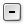Subtraction (A-B)
Maths  >  Operators  >  Subtraction
Mathematical subtraction
Inputs
A (A)First operand for subtraction
B (B)Second operand for subtraction
Outputs
Result (R)Result of subtraction
component and connecting the initial value to input A. At input B, we need a Panel with 1 (use Canvas search with "1). Now we connect output R of Subtraction with the inputs Ex and Ey of the second grid.

#### 4

##### Generate the diagonals

The component SquareSquare (SqGrid)
Vector  >  Grid  >  Square
2D grid with square cells
Inputs
Plane (P)Base plane for grid
Size (S)Size of grid cells
Extent X (Ex)Number of grid cells in base plane x direction
Extent Y (Ey)Number of grid cells in base plane y direction
Outputs
Cells (C)Grid cell outlines
Points (P)Points at grid corners
has two outputs: At S we get the outlines of the cells, in this case squares, and at P we get the points at the grid corners. The data is arranged in data tree structure
: Each branch represents a row of the grid and contains a list of squares or points.

We create the diagonals of the space frame by connecting the points of the upper grid with those of the lower grid. Such a connection can be created with the component Line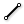Line (Ln)
Curve  >  Primitive  >  Line
Create a line between two points.
Inputs
Start Point (A)Line start point
End Point (B)Line end point
Outputs
Line (L)Line segment
. The big question is how to sort the points for the inputs A and B to create the desired connections. Let’s think of an half-octahedron (which is a pyramid) in isolation: It has a square base and an apex. The sloping edges connect the vertices of the base with the apex. The edges represent the diagonals that we are looking for. So we keep in mind: There are four vertices opposed by one vertex.

The edges of the base are the grid cell outlines at output C of Square. We can use the component Discontinuity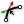Discontinuity (Disc)
Curve  >  Analysis  >  Discontinuity
Find all discontinuities along a curve.
Inputs
Curve (C)Curve to analyze
Level (L)Level of discontinuity to test for (1=C1, 2=C2, 3=Cinfinite)
Outputs
Points (P)Points at discontinuities
Parameters (t)Curve parameters at discontinuities
to generate points at all kinks of the outlines; connect output C with input C. We now have the four vertices for the base. If we take a closer look at output P, we notice that the points are sorted as a data tree, whose last ramifications contains the 4 vertices of each grid cell as a list.

Now, we have the four base vertices but the counterpart, the apex, is still missing. It’s important that the apex needs do be in the same data tree structure as our base vertices: the last ramification should contain a list with only a single vertex. In this case, we need to use the component Graft Tree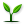Graft Tree (Graft)
Sets  >  Tree  >  Graft Tree
Graft a data tree by adding an extra branch for every item.
Inputs
Tree (T)Data tree to graft
Outputs
Tree (T)Grafted data tree
in combination with the grid corners of the lower grid: Run a wire from output P of the lower grid to the input T which grafts our data tree. We then connect both data trees with the component LineLine (Ln)
Curve  >  Primitive  >  Line
Create a line between two points.
Inputs
Start Point (A)Line start point
End Point (B)Line end point
Outputs
Line (L)Line segment
to generate the diagonals of our space frame.

#### 5

##### Construct tubes

The last step is to turn the axes into tubes. For this, we split the outlines of our cells (squares) into individual lines; the component Explode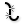Explode (Explode)
Curve  >  Util  >  Explode
Explode a curve into smaller segments.
Inputs
Curve (C)Curve to explode
Recursive (R)Recursive decomposition until all segments are atomic
Outputs
Segments (S)Exploded segments that make up the base curve
Vertices (V)Vertices of the exploded segments
will do this for us. Also, let’s not mind the doublicate lines that are caused by adjacent cells. At output S of Explode we find the separated lines and connect them together with the diagonals to input C of a Pipe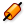Pipe (Pipe)
Surface  >  Freeform  >  Pipe
Create a pipe surface around a rail curve.
Inputs
Curve (C)Base curve
Caps (E)Specifies the type of caps (0=None, 1=Flat, 2=Round)
Outputs
Pipe (P)Resulting Pipe
component.
To connect multiple wires to one input, keep holding Shift while connecting the wires.
To connect multiple wires to one input, keep holding Shift while connecting the wires. To define a radius for the pipes, we use a PanelPanel
Params  >  Input  >  Panel
A panel for custom notes and text values
with 0.05 and connect it to input R. This will give our space frame roof some volume for a better visualization.

## Get the results

### Version Info

• Rhino 6.30
• Grasshopper 1.0.0007

As it’s often the case with writing algorithms, there are several ways to get the same solution. In this case, instead of using two square grids and connecting their vertices, the space frame roof could also be generated by creating the appropriate polyhedra and using their edges for the space frame. This is now your task! (Remember the reference to the pyramid?)

Hint 1

### Find an appropriate polyhedron

The polyhedron that we are looking is a half-octahedron, which could also be described as a pyramid. Unlike in Rhino, we can’t create them directly in Grasshopper (at least not without an external plugin). Either we import the desired geometry from Rhino to Grasshopper

or we use the component Extrude Point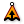Extrude Point (Extr)
Surface  >  Freeform  >  Extrude Point
Extrude curves and surfaces to a point.
Inputs
Base (B)Profile curve or surface
Point (P)Extrusion tip
Outputs
Extrusion (E)Extrusion result
in combination with a square. Let’s crate the square then.

Hint 2

### Generate the squares

The squares are created with the component SquareSquare (SqGrid)
Vector  >  Grid  >  Square
2D grid with square cells
Inputs
Plane (P)Base plane for grid
Size (S)Size of grid cells
Extent X (Ex)Number of grid cells in base plane x direction
Extent Y (Ey)Number of grid cells in base plane y direction
Outputs
Cells (C)Grid cell outlines
Points (P)Points at grid corners
, just as in the main exercise. In order for Extrude PointExtrude Point (Extr)
Surface  >  Freeform  >  Extrude Point
Extrude curves and surfaces to a point.
Inputs
Base (B)Profile curve or surface
Point (P)Extrusion tip
Outputs
Extrusion (E)Extrusion result
to work, we still need the apices of the pyramids.

Hint 3

### Create the apices

For the apices, we use the component Area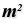Area (Area)
Surface  >  Analysis  >  Area
Solve area properties for breps, meshes and planar closed curves.
Inputs
Geometry (G)Brep, mesh or planar closed curve for area computation
Outputs
Area (A)Area of geometry
Centroid (C)Area centroid of geometry
to find the center of each grid cell and then we use Move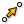Move (Move)
Transform  >  Euclidean  >  Move
Translate (move) an object along a vector.
Inputs
Geometry (G)Base geometry
Motion (T)Translation vector
Outputs
Geometry (G)Translated geometry
Transform (X)Transformation data
to move them onto the other plane. Move requires a translation vector, which we get with the component Unit Z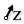Unit Z (Z)
Vector  >  Vector  >  Unit Z
Unit vector parallel to the world {z} axis.
Inputs
Factor (F)Unit multiplication
Outputs
Unit vector (V)World {z} vector
. Now we have pyramids (Breps) and only the axes for the tubes are missing.

Hint 4

### Find all lines of the space frame

The component Brep Wireframe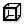Brep Wireframe (Wires)
Surface  >  Analysis  >  Brep Wireframe
Extract the wireframe curves of a brep.
Inputs
Brep (B)Base Brep
Density (D)Wireframe isocurve density
Outputs
Wireframe (W)Wireframe curves
will export the upper grid and the diagonals from the extruded Breps. For the lower grid we have to take a small detour via PolyLine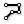PolyLine (PLine)
Curve  >  Spline  >  PolyLine
Create a polyline connecting a number of points.
Inputs
Vertices (V)Polyline vertex points
Closed (C)Close polyline
Outputs
Polyline (Pl)Resulting polyline
and connect it with the moved points. Since this only gives us one direction of the lower grid, we use PolyLinePolyLine (PLine)
Curve  >  Spline  >  PolyLine
Create a polyline connecting a number of points.
Inputs
Vertices (V)Polyline vertex points
Closed (C)Close polyline
Outputs
Polyline (Pl)Resulting polyline
again, but this time we place a Flip Matrix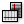Flip Matrix (Flip)
Sets  >  Tree  >  Flip Matrix
Flip a matrix-like data tree by swapping rows and columns.
Inputs
Data (D)Data matrix to flip
Outputs
Data (D)Flipped data matrix
component in front of it.

Get the results

## Get the results

### Version Info

• Rhino 6.30
• Grasshopper 1.0.0007